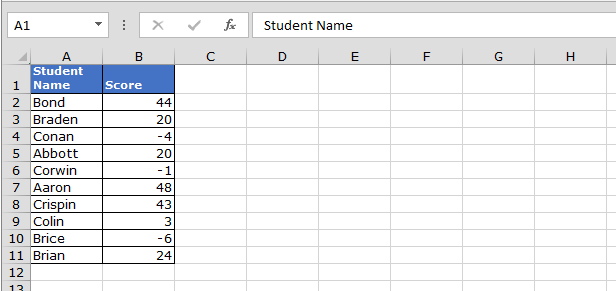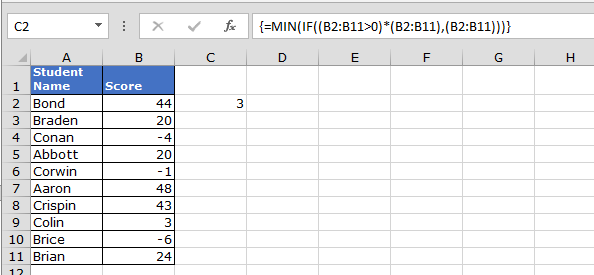# Find the minimum positive value in Microsoft Excel

In this article, we are going to learn how to find the minimum positive value in a range in Microsoft Excel. To return the positive minimum value from the data, we will use Min function along with IF function.

Why we will use Min function along with IF function?

Minimum function will help to find out the minimum number in the range. And ‘if function’ will help to pick the positive value from a range.

Let’s take an Example and understand.

We have a student list in the sheet in which we have scores, and the scores can be negative also, so we want to return minimum value from the positive values.Follow below steps to find out the minimum positive number in the range:-

• Enter the formula in cell C2.
• =MIN(IF((B2:B11>0)*(B2:B11),(B2:B11)))
• Press Ctrl+Shift+Enter.
• The formula is an Array Formula, after entering the formula in the cell, press F2 and then press Ctrl+Shift+Enter.In Excel, we can use the combination of functions to get any kind of result. Excel has  ability to save our time.We would love to hear from you, do let us know how we can improve, complement or innovate our work and make it better for you. Write us at info@exceltip.com

1."When you declare the variable, you need to state what it is, not its value:

To keep it simple, try this:

Sub Alan()

Dim N As Integer

N = 9

ActiveCell.FormulaR1C1 = ""=SUM(RC:RC["" & N & ""])""

End Sub

Note that this will write over whatever was already in the active cell as written!
"

2."the following sums next 7 columns on the right
ActiveCell.FormulaR1C1 = ""=SUM(RC:RC)""

How do I sum next N columns on the right?
dim N=9
ActiveCell.FormulaR1C1 = ""=SUM(RC:RC[&N&])"" 'will not work "

3."When you declare the variable, you need to state what it is, not its value:

To keep it simple, try this:

Sub Alan()

Dim N As Integer

N = 9

ActiveCell.FormulaR1C1 = ""=SUM(RC:RC["" & N & ""])""

End Sub

Note that this will write over whatever was already in the active cell as written!

Hope that works, "

4."the following sums next 7 columns on the right
ActiveCell.FormulaR1C1 = ""=SUM(RC:RC)""

How do I sum next N columns on the right?
dim N=9
ActiveCell.FormulaR1C1 = ""=SUM(RC:RC[&N&])"" 'will not work "

Terms and Conditions of use

The applications/code on this site are distributed as is and without warranties or liability. In no event shall the owner of the copyrights, or the authors of the applications/code be liable for any loss of profit, any problems or any damage resulting from the use or evaluation of the applications/code.